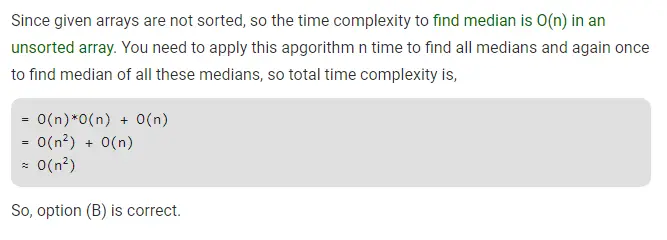# Gate CS-2019 Question Paper With Solutions

Q. 46 There are n unsorted arrays: A1, A2, ….,An. Assume that n is odd. Each of A1, A2, …., An contains n distinct elements. There are no common elements between any two arrays. The worst-case time complexity of computing the median of the medians of A1, A2, ….,An is ________ .

(A) Ο(n log n)

(B) Ο(n2)

(C) Ο(n)

(D) Ω(n2log n)